41.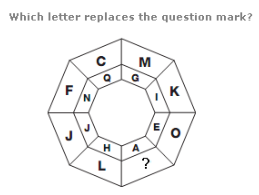A. U B. Y C. D D. S

Explanation: :

Taking pairs of letters in the inner and outer segments of each eighth of the diagram, the numerical values always add up to 20.

42.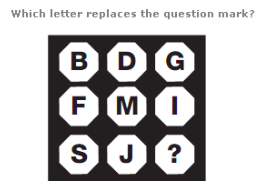A. N B. O C. L D. K

Explanation: :

Working in columns, add together the numerical values of the first and second letters, and put the letter with the reverse alphabetical value of this sum in the bottom space.

43.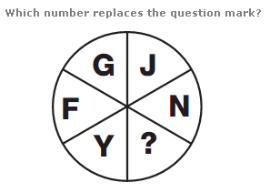A. T B. N C. S D. L

Explanation: :

Starting with the G, move clockwise around the circle, in steps of 3 letters, then 4, 5, 6 and 7.

44.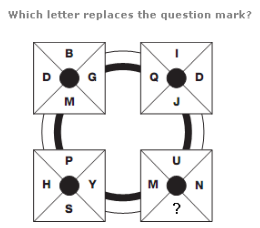A. R B. F C. E D. W

Explanation: :

Starting with the top segment of the top left square and moving clockwise around the other 3 squares, letters move through the alphabet in steps of 2, with the positions moving one place clockwise each time.

Letters in the next segment of the top left square follow the same pattern, in steps of 3 letters etc.

45.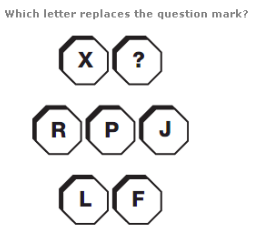A. U B. J C. S D. D